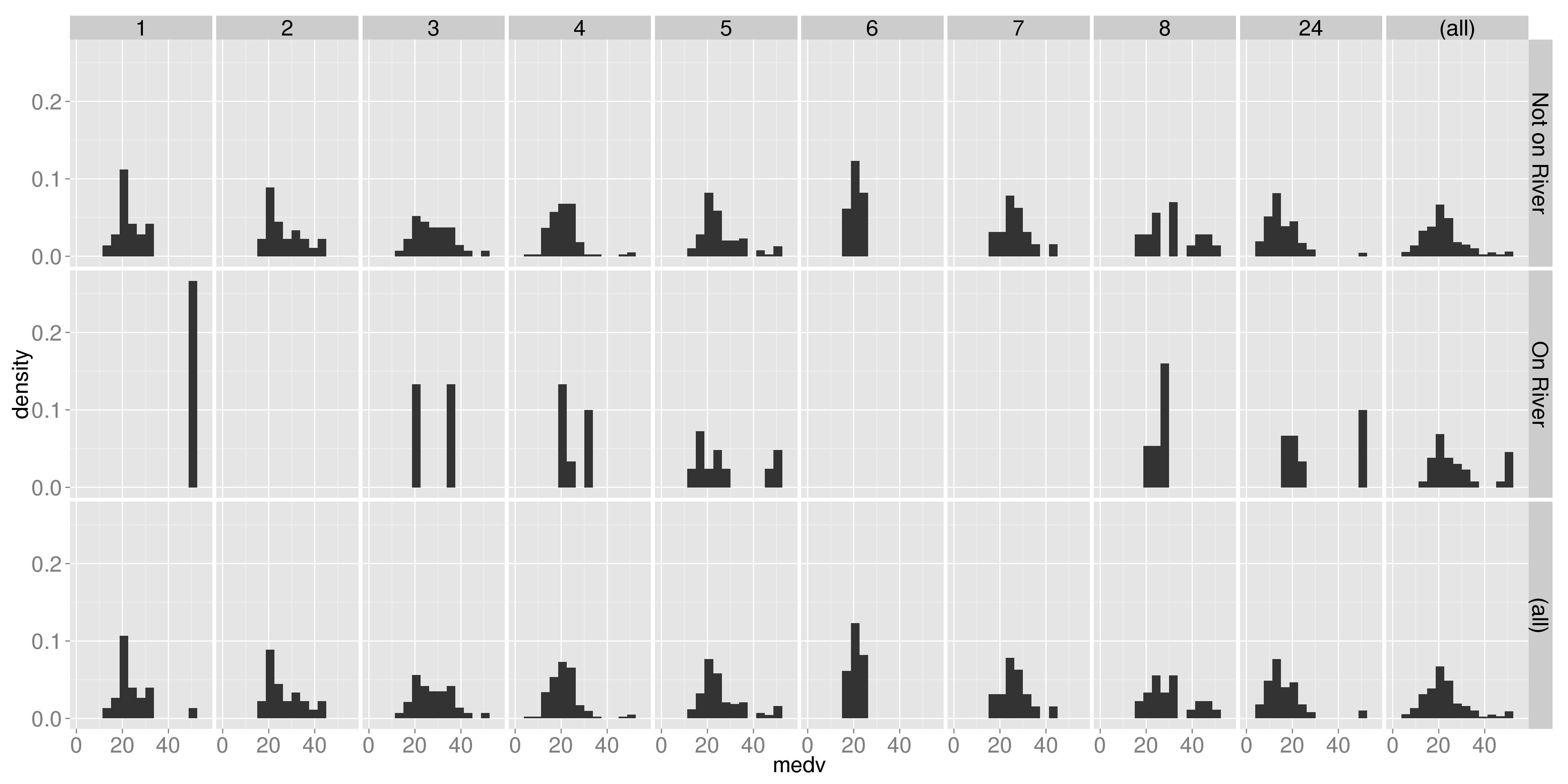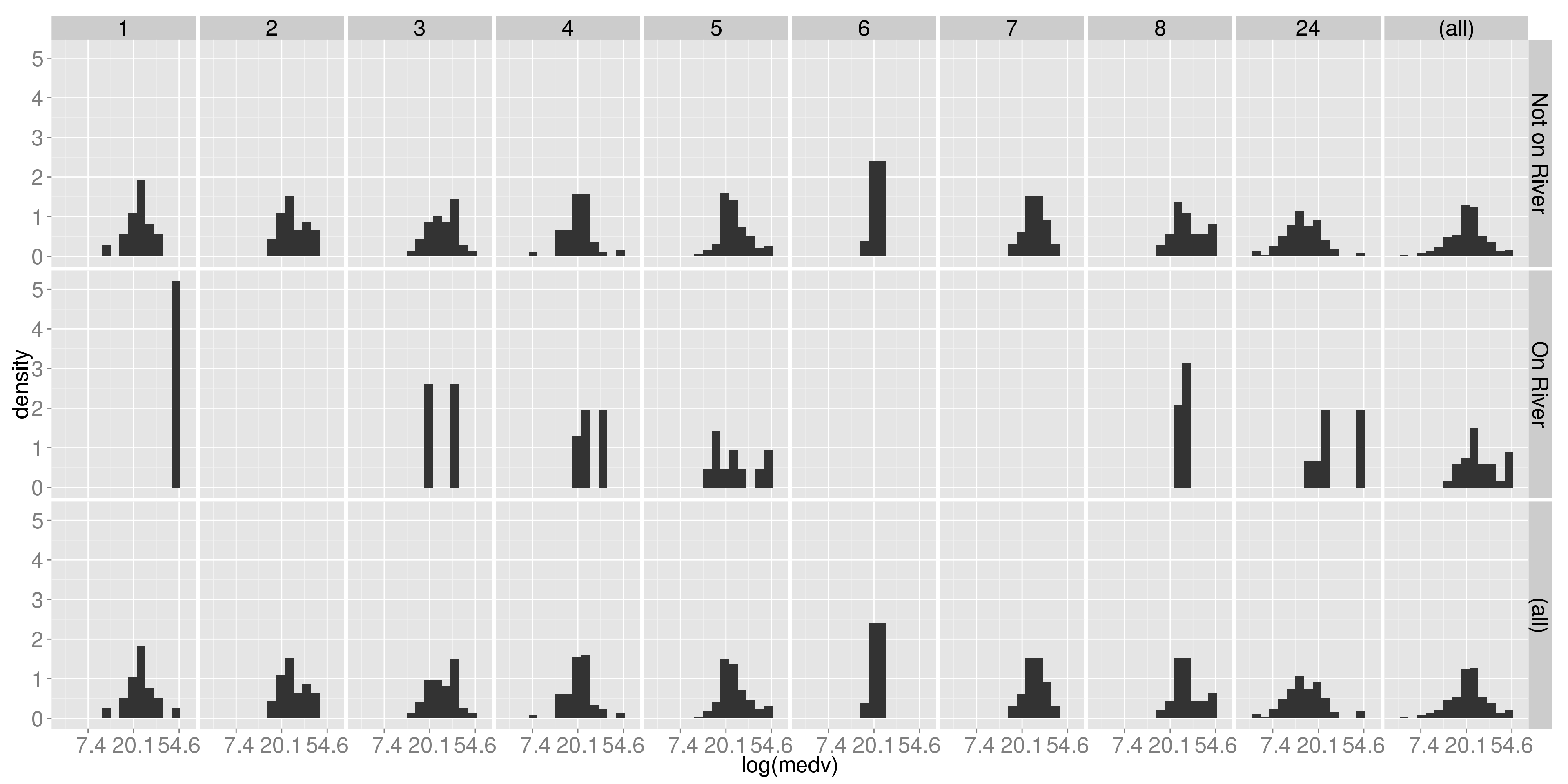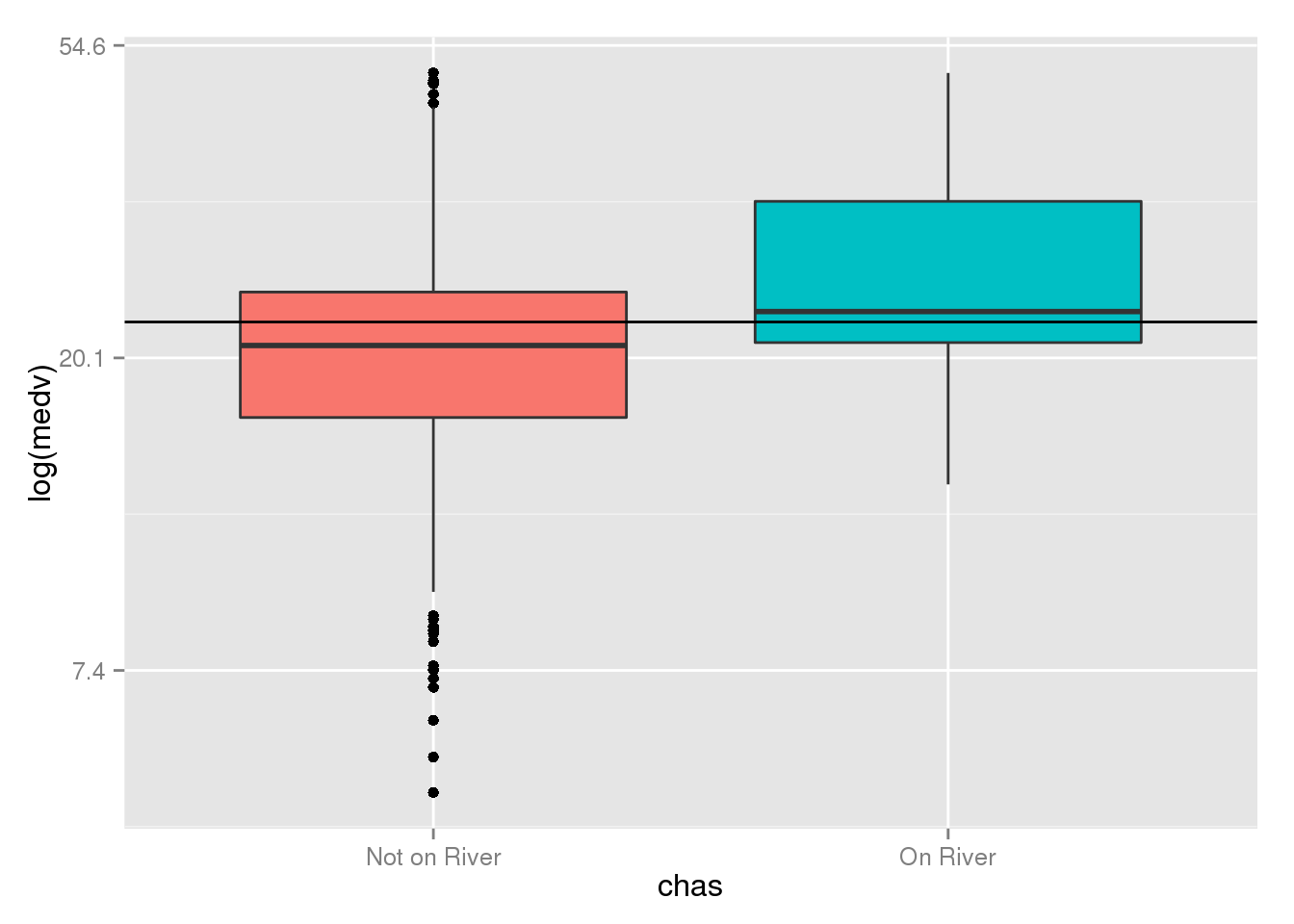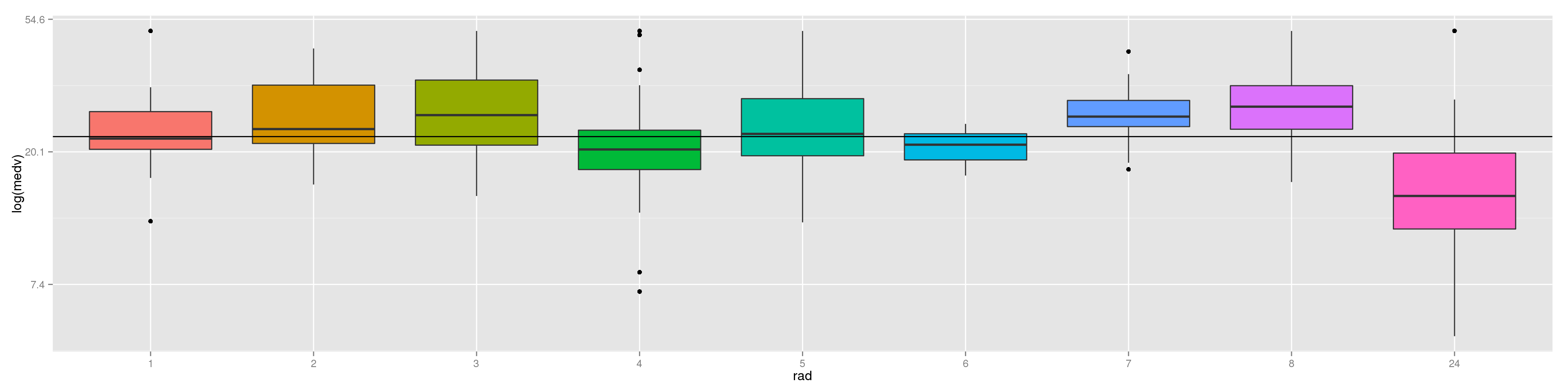# Overview

Graphically analyze using `ggplot2` the 1970 Boston housing dataset. This dataset consists of 14 variables with 506 observations. The target variable, `medv`, is the median owner-occupied home value in 1000’s of dollars. The Boston dataset is found in the `MASS` package.

``````library(MASS)
data(Boston)
Boston\$chas <-factor(Boston\$chas, levels = c(0,1),
labels = c("Not on River", "On River"))
``````##      crim zn indus         chas   nox    rm  age    dis rad tax ptratio
## 1 0.00632 18  2.31 Not on River 0.538 6.575 65.2 4.0900   1 296    15.3
## 2 0.02731  0  7.07 Not on River 0.469 6.421 78.9 4.9671   2 242    17.8
## 3 0.02729  0  7.07 Not on River 0.469 7.185 61.1 4.9671   2 242    17.8
## 4 0.03237  0  2.18 Not on River 0.458 6.998 45.8 6.0622   3 222    18.7
## 5 0.06905  0  2.18 Not on River 0.458 7.147 54.2 6.0622   3 222    18.7
## 6 0.02985  0  2.18 Not on River 0.458 6.430 58.7 6.0622   3 222    18.7
##    black lstat medv
## 1 396.90  4.98 24.0
## 2 396.90  9.14 21.6
## 3 392.83  4.03 34.7
## 4 394.63  2.94 33.4
## 5 396.90  5.33 36.2
## 6 394.12  5.21 28.7``````

Fit a linear model to `log(medv)` using select predictors.

``````attach(Boston)
Boston.lm <- lm(log(medv) ~ crim + chas + rad + lstat)
summary(Boston.lm)``````
``````##
## Call:
## lm(formula = log(medv) ~ crim + chas + rad + lstat)
##
## Residuals:
##      Min       1Q   Median       3Q      Max
## -0.64352 -0.13733 -0.02115  0.10461  0.87218
##
## Coefficients:
##               Estimate Std. Error t value Pr(>|t|)
## (Intercept)   3.428419   0.051028  67.187  < 2e-16 ***
## crim         -0.008905   0.001515  -5.876 7.73e-09 ***
## chasOn River  0.169177   0.039400   4.294 2.11e-05 ***
## rad2          0.214589   0.067157   3.195 0.001486 **
## rad3          0.204713   0.061187   3.346 0.000883 ***
## rad4          0.056412   0.054380   1.037 0.300072
## rad5          0.167914   0.053908   3.115 0.001948 **
## rad6          0.091301   0.066334   1.376 0.169330
## rad7          0.158928   0.073020   2.177 0.029990 *
## rad8          0.216818   0.067290   3.222 0.001356 **
## rad24         0.094849   0.058213   1.629 0.103879
## lstat        -0.039079   0.001667 -23.443  < 2e-16 ***
## ---
## Signif. codes:  0 '***' 0.001 '**' 0.01 '*' 0.05 '.' 0.1 ' ' 1
##
## Residual standard error: 0.2213 on 494 degrees of freedom
## Multiple R-squared:  0.7134, Adjusted R-squared:  0.707
## F-statistic: 111.8 on 11 and 494 DF,  p-value: < 2.2e-16``````

All variables are highly significant.

See the variable descriptions using `help` in the `MASS` package.

### 1. Plot and discuss the distributions of the log of the median home value (`log(medv)`) faceted by both Charles River (`chas`) and radial highway location (`rad`). Does the log transformation inprove symmetry?

``````library(ggplot2); library(scales)
## constant
f.size <- element_text(size=20)
this.theme <- theme(strip.text.x = f.size, strip.text.y = f.size,
axis.text.x = f.size, axis.text.y = f.size,
axis.title.x = f.size, axis.title.y = f.size)
b.width <- function(x) sum(c(-1, 1) * range(x))/12
medv.hist <- ggplot(Boston, aes(medv)) +
this.theme
## plot
medv.hist + geom_histogram(aes(y = ..density..), binwidth=b.width(medv))``````We can see that the median home prices are (mostly) centered around \$20,000 across radial highway scores or whether they’re in riverfront neighborhoods.

As the accessibility of highways increases, we do tend to see more right skew, suggesting that proximity to major highways raises the value of the homes. The one exception being that having a radial index of 24 lowers the price of the bulk of the homes. This may indicate that the homes with access to the most radial highways are the inner-city, which could be confounded with low-income housing.

The fact that there are few riverfront towns (only 36) makes patterns hard to detect within the group, but on the aggregate we can clearly see that having a house in a riverfront neighborhood does introduce higher home values.

Having two large peaks in the riverfront homes also suggests that we have two main populations of riverfront property – a poor and a rich section.

As we should expect from financial data, we do see right skew in all groups, or at least those with enough members to give the histogram some resolution.

``````fmt <- function(x) format(x, nsmall=1, digits=2)
medv.hist + geom_histogram(aes(y = ..density..), binwidth=b.width(log(medv))) +
scale_x_continuous(trans=log_trans(), name="log(medv)", labels = fmt) +
this.theme                 ``````As is typically seen, using a log-transform does give us mostly symmetric distributions. Our overall patterns, however, remain intact. Increasing the number of close radial highways generally raises the home value (until you have too many), as does being close to the river.

### 2. Plot and discuss the boxplot distributions of the log of the median home values (`log(medv)`) by the Charles River (`chas`) and separately by radial highway locations (`rad`). Draw horizontal lines at `mean(log(medv))` in each plot.

``````plot.box <- function(box){
box +
geom_boxplot() +
scale_y_continuous(trans=log_trans(), name="log(medv)", labels=fmt) +
geom_hline(yintercept=mean(medv)) +
theme(legend.position="none")
}
plot.box(ggplot(Boston, aes(x=chas, y=medv, fill=chas)))``````The distribution of the log-transformed median prices for riverfront homes is higher than that of other properties and still skewed slightly to the right.

The non-riverfront properties have more outliers, especially on the low side, giving us a slight left skew.

``plot.box(ggplot(Boston, aes(x=rad, y=medv, fill=rad)))``As we saw in the histograms, increasing the radial highway index generally increases the median home value. However, the boxplots now reveal a more subtle pattern – there are two distinct increasing trends: from 1 to 3 and from 4 to 8. If the two groups are considered as a whole, we see no overall trend.

We also see that the distribution of median home values is lowest for homes with an index of 24. This group also has the most variability. Again, this may point to median house prices in towns in a city center being confounded with income.

### 3. Explore graphically the relationship of crime (`crim`) to the log of the median home values (`log(medv)`) with and without faceting on the radial highway locations (`rad`). What difficulties do you see in using `crim` as a predictor?

``(gp <- ggplot(Boston, aes(y=log(medv), x=crim)) + geom_point() + this.theme)``